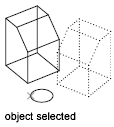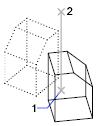# ROTATE3D (Command)

Moves objects about a 3D axis.

It is recommended that you use the gizmos available through the 3DMOVE and 3DROTATE commands to manipulate 3D objects.

The following prompts are displayed.### Select objects

Specifies which objects to rotate.

### First point on axis

Specifies the first of two points of an axis of rotation for the selected object.

Second point on axis
Specifies a second point that establishes an axis of rotation.

### Rotation angle

Specifies an angle that represents how far the object is rotated about the axis.

### Reference

Specifies the reference angle and the new angle. The difference between the starting angle and the ending angle is the computed rotation angle.

### Define axis by

Specifies a method for defining the axis of rotation.

Object
Aligns the axis of rotation with an existing object. You select one of the following object types:
• Line. Aligns the axis of rotation with a selected line.
• Circle. Aligns the axis of rotation with the 3D axis of the circle (perpendicular to the plane of the circle and passing through the center of the circle).• Arc. Aligns the axis of rotation with the 3D axis of the arc (perpendicular to the plane of the arc and passing through the center of the arc).
2D polyline segment
Aligns the axis of rotation with a segment of the polyline. Treats a straight segment as a line segment. Treats an arc segment as an arc.
Last
Uses the last axis of rotation.
View
Aligns the axis of rotation with the viewing direction of the current viewport that passes through the selected point.
X axis, Y axis, Z axis
Aligns the axis of rotation with one of the axes (X, Y, or Z) that pass through the selected point.
2 points
Uses two points to define the axis of rotation.